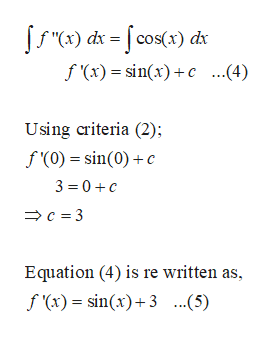# Find the function F that satisfies the following differential equations and initial conditions.F''(x)=cos x, F'(0)=3, F(pi)=5

Question
8 views

Find the function F that satisfies the following differential equations and initial conditions.

F''(x)=cos x, F'(0)=3, F(pi)=5

check_circle

Step 1

Given that,

(1) f\'\'(x)=cos x

(2) f\'(0)=3

(3)f(π)=5

Integrate equation 1;

...help_outlineImage Transcriptionclosef (x)sinx)c ...4) Using criteria (2); f(0) sin(0)c 3 0C C3 Equation (4) is re written as, f (x) sin(x)3..5) fullscreen

### Want to see the full answer?

See Solution

#### Want to see this answer and more?

Solutions are written by subject experts who are available 24/7. Questions are typically answered within 1 hour.*

See Solution
*Response times may vary by subject and question.
Tagged in

### Derivative